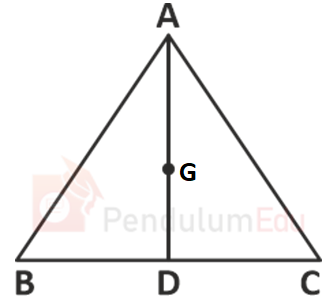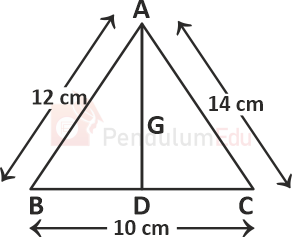Question of The Day22-11-2021

In △ ABC, G is the centroid, AB = 12 cm, BC = 10 cm and CA = 14 cm, then what is the value (in cm) of GD?Correct Answer : c ) 8 cm

Explanation :

According to the question

AB = c = 12 cm, BC = a = 10 cm and CA = b = 14 cm

We know that

median=$${1 \over 2}{\sqrt{ 2b^2+2c^2-a^2}}$$Median=$${1 \over 2}{\sqrt{ 2(12)^2+2(14)^2-(10)^2}}$$

⇒Median=$${1 \over 2}{\sqrt{ 288+392-100}}$$

Since, $${\sqrt {580}}$$ ≅ 24

⇒Median=$${1\over 2}*{\sqrt {580}}$$= $${1\over 2}*24$$

⇒ 12 cm

Centroid divides Median in the ratio 2 : 1

⇒GD=$${1 \over 3}*12$$

⇒ GD = 4 cm

Hence, (c) is the correct answer.0